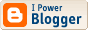## Johnny Ball: A Betrayal of Science

Last night, I took my family to see a Christmas lecture by Johnny Ball, famous as a television presenter that popularized mathematics with shows in the 1970s and 80s such as Think of a Number.

The beginning of his talk was a beautiful introduction to square laws in the work of Galileo, Kepler, and Newton, with some lovely audience-participation demonstrations showing the value of experiment. Unfortunately, this was belied by the second half, a rant in denial of climate change that included statements such as 'The greens don't want modern society to work so they've decided to demonize carbon' and 'Carbon makes up only 1/3000 of the gases in the atmosphere. Can that have such a big effect? I don't think so'. No mention of the IPCC or the scientific consensus that contradicts his view.

The danger in this is that he may leave youngsters feeling that scientific disputes are similar to political disputes, with no understanding of how the scientific method can establish truth independent of popular opinion.

I gather his hosts, the University of Edinburgh and the Royal Society of Edinburgh, had no idea he was going to go off on this tangent. Going by the first half of his talk, he used to be capable of giving a truly wonderful introduction to science for young people. But now he should not be let near them; he has betrayed his trust.

[Update: This incident was reported in the TES
Johnny causes storm in lecture on climate change.]

## Speculations on the Future of Science

From Kevin Kelly, former editor of Wired.
"Technology is, in its essence, new ways of thinking. The most powerful type of technology, sometimes called enabling technology, is a thought incarnate which enables new knowledge to find and develop news ways to know. This kind of recursive bootstrapping is how science evolves. As in every type of knowledge, it accrues layers of self-reference to its former state."

## Droste"The Droste effect is a Dutch term for a specific kind of recursive picture, one that in heraldry is termed mise en abyme."

## Arithmetic for lists

Here are analogues of sum, product, and exponentiation for lists. Each takes two lists of values, of types a and b, and forms the list of all values of sum, product, and function (exponent) types.
`type Sum a b = Either a btype Prod a b = (a,b)type Exp a b = [(b,a)] -- represents functions from b to a(+++) :: [a] -> [b] -> [Sum a b]xs +++ ys = map Left xs ++ map Right ys(***) :: [a] -> [b] -> [Prod a b]xs *** ys = [ (x,y) | x <- xs, y <- ys ](^^^) :: [a] -> [b] -> [Exp a b]xs ^^^ [] = [ [] ]xs ^^^ (y:ys) = [ (y,x):e | x <- xs, e <- xs^^^ys ]`
The first two of these are already built-in to Haskell, in the form of append and list-comprehensions. The third is also quite useful, and I suggest it should be added to the standard List module. For example, if you want to form a truth table for the list of variables in names, your can do so using [True,False]^^^names to form all 2^n mappings of names to truth variables (where n is the length of names).

In case you doubt whether these actually correspond to sum, product, and exponentiation, here are Peano's definitions:
`m+0 = mm+(n+1) = (m+n)+1m*0 = 0m*(n+1) = (m*n)+mm^0 = 1m^(n+1) = (m^n)*m`
And here are the above operations, rewritten to correspond to Peano's definitions:
`(+++) :: [a] -> [b] -> [Sum a b][] +++ ys = map Right ys(x:xs) +++ ys = Left x : (xs +++ ys)(***) :: [a] -> [b] -> [Prod a b][] *** ys = [](x:xs) *** ys = map f (ys +++ (xs *** ys))  where  f (Left y) = (x,y)  f (Right p) = p(^^^) :: [a] -> [b] -> [Exp a b]xs ^^^ [] = [ [] ]xs ^^^ (y:ys) = map g (xs *** (xs ^^^ ys))  where  g (x,e) = (y,x):e`

## Lambdacats